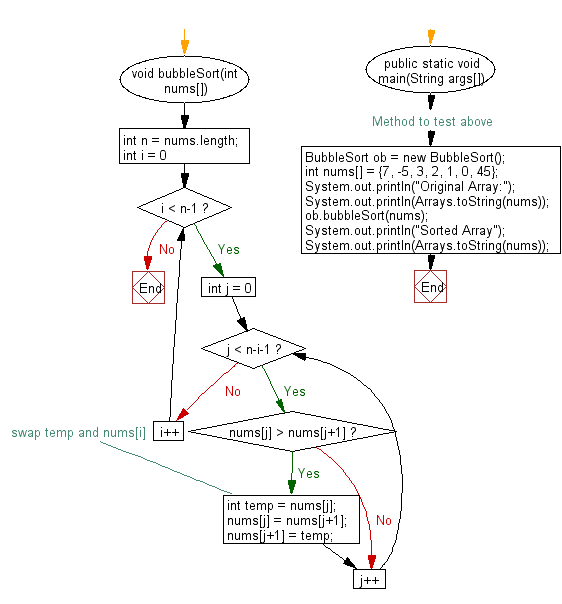﻿ Java exercises: Bubble sort Algorithm - w3resource# Java Exercises: Bubble sort Algorithm

## Java Sorting Algorithm: Exercise-2 with Solution

Write a Java program to sort an array of given integers using the Bubble sorting Algorithm.

According to Wikipedia "Bubble sort, sometimes referred to as sinking sort, is a simple sorting algorithm that repeatedly steps through the list to be sorted, compares each pair of adjacent items and swaps them if they are in the wrong order. The pass through the list is repeated until no swaps are needed, which indicates that the list is sorted. The algorithm, which is a comparison sort, is named for the way smaller elements "bubble" to the top of the list. Although the algorithm is simple, it is too slow and impractical for most problems even when compared to insertion sort. It can be practical if the input is usually in sort order but may occasionally have some out-of-order elements nearly in position."

Sample Solution:

Java Code:

``````import java.util.Arrays;
class BubbleSort
{
void bubbleSort(int nums[])
{
int n = nums.length;
for (int i = 0; i < n-1; i++)
for (int j = 0; j < n-i-1; j++)
if (nums[j] > nums[j+1])
{
// swap temp and nums[i]
int temp = nums[j];
nums[j] = nums[j+1];
nums[j+1] = temp;
}
}

// Method to test above
public static void main(String args[])
{
BubbleSort ob = new BubbleSort();
int nums[] = {7, -5, 3, 2, 1, 0, 45};
System.out.println("Original Array:");
System.out.println(Arrays.toString(nums));
ob.bubbleSort(nums);
System.out.println("Sorted Array");
System.out.println(Arrays.toString(nums));
}
}
```
```

Sample Output:

```Original Array:
[7, -5, 3, 2, 1, 0, 45]
Sorted Array
[-5, 0, 1, 2, 3, 7, 45]
```

Flowchart:Java Code Editor:

What is the difficulty level of this exercise?

﻿

## Java: Tips of the Day

Array vs ArrayLists:

The main difference between these two is that an Array is of fixed size so once you have created an Array you cannot change it but the ArrayList is not of fixed size. You can create instances of ArrayLists without specifying its size. So if you create such instances of an ArrayList without specifying its size Java will create an instance of an ArrayList of default size.

Once an ArrayList is full it re-sizes itself. In fact, an ArrayList is internally supported by an array. So when an ArrayList is resized it will slow down its performance a bit as the contents of the old Array must be copied to a new Array.

At the same time, it's compulsory to specify the size of an Array directly or indirectly while creating it. And also Arrays can store both primitives and objects while ArrayLists only can store objects.

Ref: https://bit.ly/3o8L2KH